# MCQs on Electric Circuits

##### Page 45 of 63. Go to page 1 2 3 4 5 6 7 8 9 10 11 12 13 14 15 16 17 18 19 20 21 22 23 24 25 26 27 28 29 30 31 32 33 34 35 36 37 38 39 40 41 42 43 44 45 46 47 48 49 50 51 52 53 54 55 56 57 58 59 60 61 62 63
01․ The voltage across R and L in a series RL circuit are found to be 200 V and 150 V respectively. The rms value of the voltage across the series combination is __________________ V.
360.
250.
200.
450.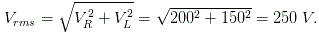02․ Whenever current is supplied by a source its terminal voltage
increases.
decreases.
remains constant.
increases exponentially.

Whenever current is supplied by a source, this current also flows through the internal resistance connected in series in the source. Because of voltage drop across the internal resistance, the terminal voltage is decreased.

03․ A current of 4 A flows in an AC circuit when 100 V DC is applied to it whereas it takes 250 V AC to produce the same current the power factor of the circuit is
0.4.
10.
1.
0.85.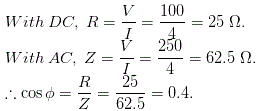04․ Which of the following can not be connected in series unless they are identical?
Voltage source.
Current source.
Both of above.
Resistance.

Current source can not be connected in series unless identical because in series all individual current sources have same values.

05․ A reactance having an inductor of 0.15 H is connected in series with 10 Ω resistance. What will be the inductive reactance?
48.15 Ω.
47.1 Ω.
1.5 Ω.
None of these.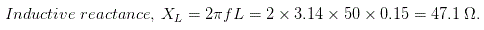06․ Kirchhoff's second law is based on law of conservation of
charge.
energy.
momentum.
mass.

Kirchhoff's voltage law (KVL) is also called Kirchhoff's second law. The principle of conservation of energy implies that the directed sum of the electrical potential differences (voltage) around any closed network is zero.

07․ A series circuit consists of R = 20 Ω, L = 20 mH, and AC supply 60 V with f = 100 Hz. The current in R is
2.54 A.
1.27 A.
5.08 A.
10.16 A.

Here, R = 20 Ω, L = 20 mH, V = 60 V and f = 100 Hz.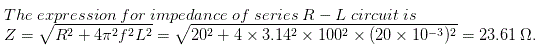Therefore, current through the circuit i.e. both through resistor and inductor will be 60 / 23.61 = 2.54 A.

08․ A series circuit consists of R = 20 Ω, L = 20 mH, and AC supply 60 V with f = 100 Hz. The voltage drop across R is
30.6 V.
50.8 V.
40.8 V.
24.4 V .

Here, R = 20 Ω, L = 20 mH, V = 60 V and f = 100 HzTherefore, current through the circuit i.e. both through resistor and inductor will be 60 / 23.61 = 2.54 A The voltage drop across R is V = IR = 2.54 X 20 V = 50.8 V.

09․ A series circuit consists of R = 20 Ω, L = 20 mH, and AC supply 60 V with f = 100 Hz. The voltage drop across L is
39.1 V.
31.9 V.
45.5 V.
50.5 V.

Here, R = 20 Ω, L = 20 mH, V = 60 V and f = 100 HzThe reactance of the circuit, X = 2πfL = 2 X 3.14 X 100 X 20 X 10 - 3 = 12.56 Ω ∴ Electric current through the circuit i.e both through resistor and inductor will be 60 / 23.61 = 2.54 A Voltage drop across L is XI = 12.56 X 2.54 = 31.9 V.

10․ A series circuit consists of R = 20 Ω, L = 20 mH, and AC supply 60 V with f = 100 Hz. The phase angle of current in respect of supply voltage will be
40.4o.
32.1o.
28.8o.
20.2o.

Here, R = 20 Ω, L = 20 mH, V = 60 V and f = 100 Hz.The reactance of the circuit, X = 2πfL = 2 × 3.14 × 100 × 20 × 10 - 3 = 12.56 Ω. ∴ The phase angle of current in respect of supply voltage will be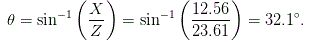<<<4344454647>>>